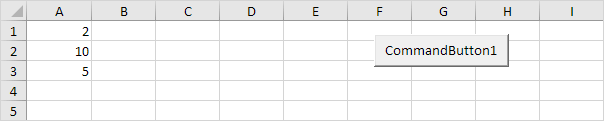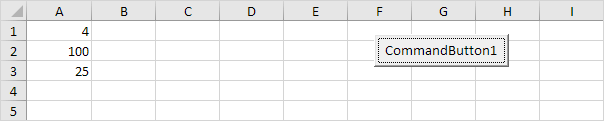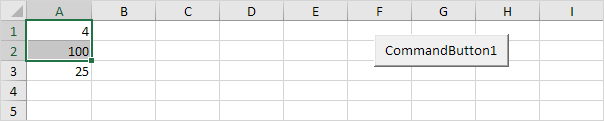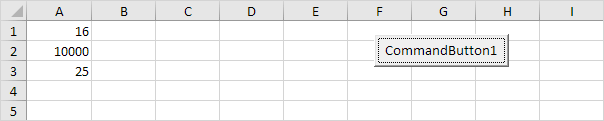# Loop through Defined Range

Below we will look at a program that loops through a defined range. For example, when we want to square the numbers in Range("A1:A3"). Did you know you can also loop through a dynamic range?

Situation:Place a command button on your worksheet and add the following code lines:

1. First, we declare two Range objects. We call the Range objects rng and cell.

Dim rng As Range, cell As Range

2. We initialize the Range object rng with Range("A1:A3").

Set rng = Range("A1:A3")

3. Add the For Each Next loop.

For Each cell In rng

Next cell

Note: rng and cell are randomly chosen here, you can use any names. Remember to refer to these names in the rest of your code.

4. Next, we square each cell in this range. To achieve this, add the following code line to the loop:

cell.Value = cell.Value * cell.Value

Result when you click the command button on the sheet:5. If you want to check each cell in a randomly selected range, simply replace:

Set rng = Range("A1:A3")

with:

Set rng = Selection

6. Now, for example select Range("A1:A2").Result when you click the command button on the sheet:Go to Next Chapter: Macro Errors